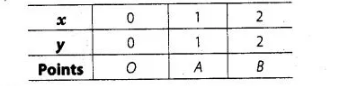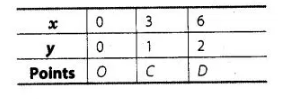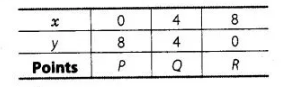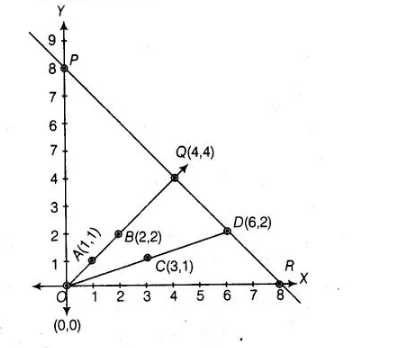# Determine graphically,

Question:

Determine graphically, the vertices of the triangle formed by the lines

y = x, 3y = x and x + y = 8

Solution:

Given linear equations are

$y=x$ ...(i)

$3 y=x$ ....(ii)

and   $x+y=8$....(iii)

For equation $y=x$

If $x=1$, then $y=1$

If $x=0$, then $y=0$

If $x=2$, then $y=2$

Table for line $y=x$,For equation $x=3 y$,

If $x=0$, then $y=0$; if $x=3$, then $y=1$ and if $x=6$, then $y=2$

Table for line $x=3 y$,For equation. $x+y=8 \Rightarrow y=8-x$

If $x=0$, then $y=8$; if $x=8$, then $y=0$ and if $x=4$, then $y=4$ Table for line $x+y=8$,

For equation. $x+y=8 \Rightarrow y=8-x$

If $x=0$, then $y=8$; if $x=8$, then $y=0$ and if $x=4$, then $y=4$ Table for line $x+y=8$,Plotting the points A(1,1) and 6(2,2), we get the straight line AB. Plotting the points C(3,1) and 0(6,2), we get the straight line CD. Plotting the

points P(0, 8), Q(4, 4) and R{8, 0), we get the straight line PQR. We see that lines AB and CD intersecting the line PR on Q and D, respectively.

So, AOQD is formed by these lines. Hence, the vertices of the A00D formed by the given lines are0(0, 0),Q(4, 4)and 0(6,2).